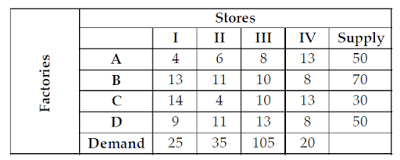A directory of Objective Type Questions covering all the Computer Science subjects. Here you can access and discuss Multiple choice questions and answers for various compitative exams and interviews.

#### Important Notice!

Dear users, compscibits.com is now permanently moved to compsciedu.com. Please update all your links and bookmarks accordingly. Soon, the site will be accessible through URL compsciedu.com only.

 101. Which of the following is used to make an Abstract class ? a. Making atleast one member function as pure virtual function b. Making atleast one member function as virtual function c. Declaring as Abstract class using virtual keyword d. Declaring as Abstract class using static keyword

 102. Match the following with reference to object oriented modelling :List - I                         List - II(a) Polymorphism        (i) Picking both operator and attributes with operations appropriate to model an object(b) Inheritance             (ii) Hiding implementation details of methods from users of objects(c) Encapsulation        (iii) Using similar operations to do similar things(d) Abstraction              (iv) Create new classes from existing classCodes :      (a)   (b)  (c)   (d) a. (iv)  (iii)   (i)   (ii) b. (iii)  (iv)   (i)   (ii) c. (iii)  (i)     (ii)  (iv) d. (iv)  (iii)   (ii)  (i)

 103. Which of the following is/are correct with reference to Abstract class and interface ?(a) A class can inherit only one Abstract class but may inherit several interfaces.(b) An Abstract class can provide complete and default code but an interface has no code. a. (a) is true b. (b) is true c. Both (a) and (b) are true d. Neither (a) nor (b) is true

 104. Consider the following conditions :(a) The solution must be feasible, i.e. it must satisfy all the supply and demand constraints.(b) The number of positive allocations must be equal to m+n-1, where m is the numberof rows and n is the number of columns.(c) All the positive allocations must be in independent positions.The initial solution of a transportation problem is said to be non-degenerate basic feasible solution if it satisfies : a. (a) and (b) only b. (a) and (c) only c. (b) and (c) only d. (a), (b) and (c)

 105. Consider the following transportation problem. The transportation cost in the initial basic feasible solution of the above transportation problem using Vogel’s Approximation method is :a. 1450 b. 1465 c. 1480 d. 1520

 106. Big-O estimates for the factorial function and the logarithm of the factorial function i.e. n! and log n! is given by a. O(n!) and O(n log n) b. O(n^n) and O(n log n) c. O(n!) and O(log n!) d. O(n^n) and O(log n!)

 107. Match the following:List-I                                       List-IIa. Classification           i. Principal Component Analysisb. Clustering                 ii. Branch and Boundc. Feature Extraction   iii. K-nearest neighbourd. Feature Selection   iv. K-meansCodes:      a    b    c    d a. iii   iv    ii     i b. iv   iii    i     ii c. iii   iv    i     ii d. iv   iii    ii    i